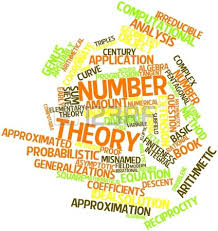# The Textbook of Number TheoryA Number Theory textbook has N pages, numbered the usual way, from 1 to N.

The total number of digits in the page numbers is 2832.

How many pages does the textbook have?

×